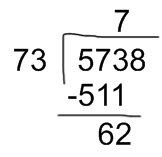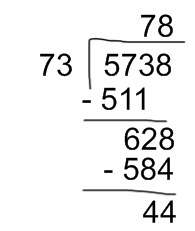Smartick is an advanced online program that teaches kids math and coding in only 15 min. a day

Aug08

# How to Do 2 and 3 Digit Division

In this post, we are going to learn how to do 2 and 3 digit division.

In order to do 2 and 3 digit division, follow these steps:

• First, take as many digits of the dividend that the divisor has. If the digits of the dividend are smaller than the divisor, take one more digit of the dividend.For example, if we want to divide 5738 / 73, the first thing we have to do is take the first two digits from the dividend, 57, but as 57 is less than 73, so we have to take another digit from the dividend; therefore, it is 573.

• Second, divide the first number of the dividend (or the first two if we have to take another digit) into the first number of the divisor and test if it divides evenly. If it does not divide evenly, test with the previous number.If we follow the previous example, we have to divide 57 by 7. Since 8 x 7 = 56, we will try with 8. We multiply 73 x 8 = 584 and since 584 is greater than 573, the 8 does not divide evenly, thus we have to try it with the previous number.

73 x 7 = 511 and since 511 is smaller than 573, the 7 divides evenly and we will be able to subtract 573 – 511 = 62

• Third, bring down the next digit and divide like in the last step until there are no more digits.Follow along with our example of division with double-digits. The next number we have to drop down is the 8. In order to do this, we must divide 628 by 73.

We take the new first two digits, 62, and we have to divide them by 7. Since 8 x 7 = 56, we write 8 in the quotient and multiply 73 x 8 = 584. As 584 is smaller than 628, we can continue on to subtracting 628 – 584 = 44. As there are not any more numbers to bring down, we have finished the division. The solution is 78 with a remainder of 44.